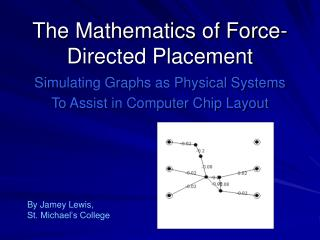DownloadDownload PresentationThe Mathematics of Force-Directed Placement

# The Mathematics of Force-Directed Placement

Download Presentation## The Mathematics of Force-Directed Placement

- - - - - - - - - - - - - - - - - - - - - - - - - - - E N D - - - - - - - - - - - - - - - - - - - - - - - - - - -
##### Presentation Transcript

1. The Mathematics of Force-Directed Placement Simulating Graphs as Physical Systems To Assist in Computer Chip Layout By Jamey Lewis, St. Michael’s College

2. How do we convert… …this… … into this?

3. The Problem • We have a graph of nodes and edges (gates and wires in the computer chip). • We need to find a good layout for the graph that provides a geometric representation of its components and makes graph symmetry clearly visible. • In industry there may be constraints on the size of the components, but they may also simply need to be far enough apart to distinguish.

4. The Solution • Simulate the graph or network as a physical model (masses and springs, for example) and subject it to physical forces. • The unnecessarily-long edges will be the most tense, and will try to contract the most. • When the nodes and edges have pushed and pulled themselves to equilibrium, we will have a geometric representation of the graph in the plane.

5. The Spring Embedder • A model of masses and springs, corresponding to nodes and edges on a graph, is called a Spring Embedder. • Spring forces exist between nodes and may attract or repel depending on how contracted they are, and on how contracted they want to be (desired/ideal length). • Electrical forces may exist to repel nodes from one another, to keep them as far apart as constraints dictate, if spring repulsion is insufficient.

6. The Spring Force The x-component of the Spring force equation on vertex v. The y-component equation mirrors this one. The force is applied to v by all springs (edges) between v and another vertex. k(1): the stiffness of the spring between the two vertices L : the resting length of the spring d(p1,p2) : the linear distance between the two vertices x : the x-coordinate of a vertex’ position

7. The Repulsive Force The x-component of the Repulsive force equation on vertex v. The y-component equation mirrors this one. The force is applied to v by all other vertices (perhaps limited to merely those within a specific effective range). k(2): electrical repulsion constant between two vertices d(p1,p2) : the linear distance between the two vertices x : the x-coordinate of a vertex’ position

8. An Example

9. Concerning the Barycentric Model • Some Spring Embedders use a Gravitational force to attract all nodes toward the graph’s Barycenter (the average of all node positions) in order to show symmetry more clearly and keep loosely-connected groups of nodes from drifting. • Others simulate this by creating at least 3 Fixed vertices on the edges of the graph and giving all edges an ideal length of 0, causing them to want to contract as long as they are able, counteracted only by the attraction from the fixed vertices.

10. My Implementation • Instead of directly implementing simulated Gravity, I chose to use the method of setting some but not all Ideal Edge Lengths to 0. • This results in a graph that is sufficiently flexible to show off geometry, yet not unnecessarily bent towards the middle to produce a circular formation. • However, I also retained the Repulsive forces that are not present in the 2nd method, so that fixed points would not be a requirement.

11. A Problem: Oscillation • One of the problems of Spring Embedder implementation is of Oscillation, that is, when nodes move back and forth repetitively without ever stabilizing. • This happens because the application of forces in a computer program occurs in steps and not continuously, and moving vertices are given the chance to move past their stable destinations in a single step, forcing them to move back and forth. • A form of oscillation that also occurs is Rotation, when vertices continuously move in a circle.

12. The Solution: Temperature • A solution to this problem is to implement a “Temperature” for each vertex, acting as a factor for its movement. Hot = fast, cold = slow. • When traveling continuously in the same direction, a Vertex’ temperature will increase and it will speed up. When Oscillation or Rotation are detected, the Vertex’ temperature will drop, and it will slow down.

13. Oscillation and Temperature The direction of the impulse of vertex v. After adjustment based on which quadrant the movement is in, this can be used to determine if the new direction is over 90 degrees different from the old direction. θ : the direction of the impulse of vertex v Δ : the change in x or y position of vertex v

14. Oscillation and Temperature The x-component of the movement of vertex v as affected by Temperature. The y-component equation mirrors this one. Δ: the change in x position of vertex v as a result of forces T : the Temperature variable of vertex v, ranging from 0-100

15. Example

16. One More Example

17. END Thanks for listening!